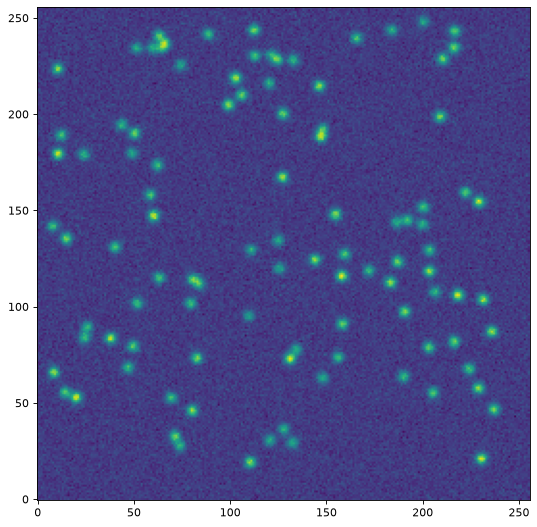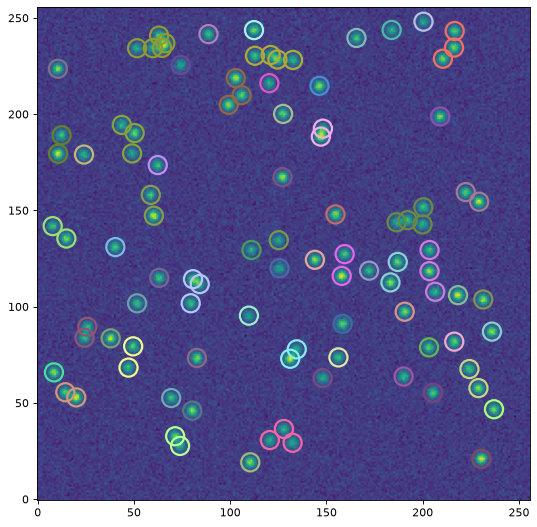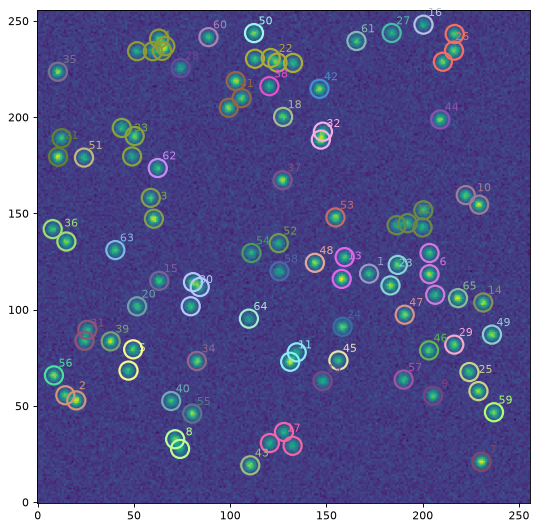# Grouping Algorithms¶

## Introduction¶

In Point Spread Function (PSF) photometry, a grouping algorithm is used to separate stars into optimum groups. The stars in each group are defined as those close enough together such that they need to be fit simultaneously, i.e. their profiles overlap.

Photoutils currently provides two classes to group stars:

## DAOPHOT GROUP¶

Stetson, in his seminal paper (Stetson 1987, PASP 99, 191), provided a simple and powerful grouping algorithm to decide whether the profile of a given star extends into the fitting region of any other star. Stetson defines this in terms of a “critical separation” parameter, which is defined as the minimal distance that any two stars must be separated by in order to be in different groups. Stetson gives intuitive reasoning to suggest that the critical separation may be defined as a multiple of the stellar full width at half maximum (FWHM).

Photutils provides an implementation of the DAOPHOT GROUP algorithm in the DAOGroup class. Let’s take a look at a simple example.

First, let’s make some Gaussian sources using make_random_gaussians_table and make_gaussian_sources_image. The former will return a Table containing parameters for 2D Gaussian sources and the latter will make an actual image using that table.

from collections import OrderedDict
import numpy as np
from photutils.datasets import (make_random_gaussians_table,
make_gaussian_sources_image)
import matplotlib.pyplot as plt

n_sources = 350
sigma_psf = 2.0

# use an OrderedDict to ensure reproducibility
params = OrderedDict([('flux', [500, 5000]),
('x_mean', [6, 250]),
('y_mean', [6, 250]),
('x_stddev', [sigma_psf, sigma_psf]),
('y_stddev', [sigma_psf, sigma_psf]),
('theta', [0, np.pi])])

starlist = make_random_gaussians_table(n_sources, params,
random_state=1234)

shape = (256, 256)
sim_image = make_gaussian_sources_image(shape, starlist)

plt.imshow(sim_image, origin='lower', interpolation='nearest',
cmap='viridis')
plt.show()starlist is an astropy Table of parameters defining the position and shape of the stars.

Next, we need to rename the table columns of the centroid positions so that they agree with the names that DAOGroup expect. Here we rename x_mean to x_0 and y_mean to y_0:

>>> starlist['x_mean'].name = 'x_0'
>>> starlist['y_mean'].name = 'y_0'


Now, let’s find the stellar groups. We start by creating a DAOGroup object. Here we set its crit_separation parameter 2.5 * fwhm, where the stellar fwhm was defined above when we created the stars as 2D Gaussians. In general one will need to measure the FWHM of the stellar profiles.

>>> from astropy.stats import gaussian_sigma_to_fwhm
>>> from photutils.psf.groupstars import DAOGroup
>>> fwhm = sigma_psf * gaussian_sigma_to_fwhm
>>> daogroup = DAOGroup(crit_separation=2.5*fwhm)


daogroup is a DAOGroup instance that can be used as a calling function that receives as input a table of stars (e.g. starlist):

>>> star_groups = daogroup(starlist)


The star_groups output is copy of the input starlist table, but with an extra column called group_id. This column contains integers that represent the group assigned to each source. Here the grouping algorithm separated the 350 stars into 92 distinct groups:

>>> print(max(star_groups['group_id']))
92


One can use the group_by functionality from Table to create groups according to group_id:

>>> star_groups = star_groups.group_by('group_id')
>>> print(star_groups)
flux          x_0           y_0      ...   amplitude    id group_id
------------- ------------- ------------- ... ------------- --- --------
1361.83752671 182.958386152 178.708228379 ... 54.1857935158   1        1
4282.41965053 179.998944123 171.437757021 ... 170.392063944 183        1
555.831417775 181.611905957  185.16181342 ... 22.1158294162 222        1
3299.48946968  243.60449392 85.8926967927 ... 131.282514695   2        2
2469.77482553 136.657577889 109.771746713 ... 98.2692179518   3        3
...           ...           ... ...           ... ...      ...
818.132804377 117.787387455 92.4349134636 ... 32.5524699806 313       88
3979.57421702  154.85279495 18.3148180315 ...  158.34222701 318       89
3622.30997136 97.0901736699 50.3565997421 ... 144.127134338 323       90
765.47561385 144.952825542 7.57086675812 ... 30.4573069401 330       91
1508.68165551 54.0404934991 232.693833605 ... 60.0285357567 349       92
Length = 350 rows


Finally, let’s plot a circular aperture around each star, where stars in the same group have the same aperture color:

>>> import numpy as np
>>> from photutils import CircularAperture
>>> from photutils.utils import make_random_cmap
>>> plt.imshow(sim_image, origin='lower', interpolation='nearest',
...            cmap='Greys_r')
>>> cmap = make_random_cmap(random_state=12345)
>>> for i, group in enumerate(star_groups.groups):
>>>     xypos = np.transpose([group['x_0'], group['y_0']])
>>>     ap = CircularAperture(xypos, r=fwhm)
>>>     ap.plot(color=cmap.colors[i])
>>> plt.show()## DBSCANGroup¶

Photutils also provides a DBSCANGroup class to group stars based on the Density-Based Spatial Clustering of Applications with Noise (DBSCAN) algorithm. DBSCANGroup provides a more general algorithm than DAOGroup.

Here’s a simple example using DBSCANGroup with min_samples=1 and metric=euclidean. With these parameters, the result is identical to the DAOGroup algorithm. Note that scikit-learn must be installed to use DBSCANGroup.## Reference/API¶

This module provides classes to perform grouping of stars.

### Classes¶

 DAOGroup(crit_separation) This class implements the DAOGROUP algorithm presented by Stetson (1987). DBSCANGroup(crit_separation[, min_samples, …]) Class to create star groups according to a distance criteria using the Density-based Spatial Clustering of Applications with Noise (DBSCAN) from scikit-learn. GroupStarsBase This base class provides the basic interface for subclasses that are capable of classifying stars in groups.